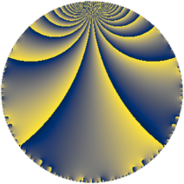Properties

 Label 342.4.gLevel $342$ Weight $4$ Character orbit 342.g Rep. character $\chi_{342}(163,\cdot)$ Character field $\Q(\zeta_{3})$ Dimension $50$ Newform subspaces $10$ Sturm bound $240$ Trace bound $5$

Related objects

Defining parameters

 Level: $$N$$ $$=$$ $$342 = 2 \cdot 3^{2} \cdot 19$$ Weight: $$k$$ $$=$$ $$4$$ Character orbit: $$[\chi]$$ $$=$$ 342.g (of order $$3$$ and degree $$2$$) Character conductor: $$\operatorname{cond}(\chi)$$ $$=$$ $$19$$ Character field: $$\Q(\zeta_{3})$$ Newform subspaces: $$10$$ Sturm bound: $$240$$ Trace bound: $$5$$ Distinguishing $$T_p$$: $$5$$

Dimensions

The following table gives the dimensions of various subspaces of $$M_{4}(342, [\chi])$$.

Total New Old
Modular forms 376 50 326
Cusp forms 344 50 294
Eisenstein series 32 0 32

Trace form

 $$50 q - 2 q^{2} - 100 q^{4} + 8 q^{5} + 60 q^{7} + 16 q^{8} + O(q^{10})$$ $$50 q - 2 q^{2} - 100 q^{4} + 8 q^{5} + 60 q^{7} + 16 q^{8} + 20 q^{10} + 134 q^{11} - 72 q^{13} - 12 q^{14} - 400 q^{16} - 12 q^{17} + 243 q^{19} - 64 q^{20} + 126 q^{22} - 46 q^{23} - 861 q^{25} + 344 q^{26} - 120 q^{28} - 80 q^{29} + 368 q^{31} - 32 q^{32} + 76 q^{34} - 444 q^{35} - 248 q^{37} - 88 q^{38} + 80 q^{40} - 959 q^{41} - 874 q^{43} - 268 q^{44} - 1584 q^{46} - 1192 q^{47} + 3578 q^{49} + 2060 q^{50} - 288 q^{52} - 964 q^{53} + 1210 q^{55} + 96 q^{56} + 424 q^{58} + 375 q^{59} - 1304 q^{61} + 280 q^{62} + 3200 q^{64} + 1780 q^{65} - 905 q^{67} + 96 q^{68} + 600 q^{70} - 580 q^{71} - 2007 q^{73} - 56 q^{74} + 252 q^{76} - 2652 q^{77} + 1706 q^{79} + 128 q^{80} + 2018 q^{82} + 5598 q^{83} + 1566 q^{85} + 424 q^{86} - 1008 q^{88} + 300 q^{89} - 256 q^{91} - 184 q^{92} - 3192 q^{94} - 4874 q^{95} - 3381 q^{97} - 2434 q^{98} + O(q^{100})$$

Decomposition of $$S_{4}^{\mathrm{new}}(342, [\chi])$$ into newform subspaces

Label Dim. $$A$$ Field CM Traces $q$-expansion
$a_{2}$ $a_{3}$ $a_{5}$ $a_{7}$
342.4.g.a $2$ $20.179$ $$\Q(\sqrt{-3})$$ None $$-2$$ $$0$$ $$-6$$ $$38$$ $$q+(-2+2\zeta_{6})q^{2}-4\zeta_{6}q^{4}+(-6+6\zeta_{6})q^{5}+\cdots$$
342.4.g.b $2$ $20.179$ $$\Q(\sqrt{-3})$$ None $$-2$$ $$0$$ $$2$$ $$-42$$ $$q+(-2+2\zeta_{6})q^{2}-4\zeta_{6}q^{4}+(2-2\zeta_{6})q^{5}+\cdots$$
342.4.g.c $2$ $20.179$ $$\Q(\sqrt{-3})$$ None $$2$$ $$0$$ $$-12$$ $$16$$ $$q+(2-2\zeta_{6})q^{2}-4\zeta_{6}q^{4}+(-12+12\zeta_{6})q^{5}+\cdots$$
342.4.g.d $2$ $20.179$ $$\Q(\sqrt{-3})$$ None $$2$$ $$0$$ $$3$$ $$-64$$ $$q+(2-2\zeta_{6})q^{2}-4\zeta_{6}q^{4}+(3-3\zeta_{6})q^{5}+\cdots$$
342.4.g.e $4$ $20.179$ $$\Q(\sqrt{-3}, \sqrt{-10})$$ None $$4$$ $$0$$ $$8$$ $$36$$ $$q+2\beta _{1}q^{2}+(-4+4\beta _{1})q^{4}+(4\beta _{1}+\beta _{2}+\cdots)q^{5}+\cdots$$
342.4.g.f $6$ $20.179$ $$\mathbb{Q}[x]/(x^{6} - \cdots)$$ None $$-6$$ $$0$$ $$1$$ $$52$$ $$q-2\beta _{4}q^{2}+(-4+4\beta _{4})q^{4}+(-\beta _{1}+\cdots)q^{5}+\cdots$$
342.4.g.g $6$ $20.179$ 6.0.6967728.1 None $$-6$$ $$0$$ $$2$$ $$-34$$ $$q+2\beta _{3}q^{2}+(-4-4\beta _{3})q^{4}+(-\beta _{3}+\cdots)q^{5}+\cdots$$
342.4.g.h $6$ $20.179$ 6.0.627014547.1 None $$6$$ $$0$$ $$10$$ $$14$$ $$q+2\beta _{1}q^{2}+(-4+4\beta _{1})q^{4}+(3\beta _{1}-\beta _{2}+\cdots)q^{5}+\cdots$$
342.4.g.i $10$ $20.179$ $$\mathbb{Q}[x]/(x^{10} - \cdots)$$ None $$-10$$ $$0$$ $$10$$ $$22$$ $$q+(-2+2\beta _{1})q^{2}-4\beta _{1}q^{4}+(2-2\beta _{1}+\cdots)q^{5}+\cdots$$
342.4.g.j $10$ $20.179$ $$\mathbb{Q}[x]/(x^{10} - \cdots)$$ None $$10$$ $$0$$ $$-10$$ $$22$$ $$q+(2-2\beta _{1})q^{2}-4\beta _{1}q^{4}+(-2+2\beta _{1}+\cdots)q^{5}+\cdots$$

Decomposition of $$S_{4}^{\mathrm{old}}(342, [\chi])$$ into lower level spaces

$$S_{4}^{\mathrm{old}}(342, [\chi]) \cong$$ $$S_{4}^{\mathrm{new}}(19, [\chi])$$$$^{\oplus 6}$$$$\oplus$$$$S_{4}^{\mathrm{new}}(38, [\chi])$$$$^{\oplus 3}$$$$\oplus$$$$S_{4}^{\mathrm{new}}(57, [\chi])$$$$^{\oplus 4}$$$$\oplus$$$$S_{4}^{\mathrm{new}}(114, [\chi])$$$$^{\oplus 2}$$$$\oplus$$$$S_{4}^{\mathrm{new}}(171, [\chi])$$$$^{\oplus 2}$$